Home

 << Previous      2    Next >>

The Sri Yantra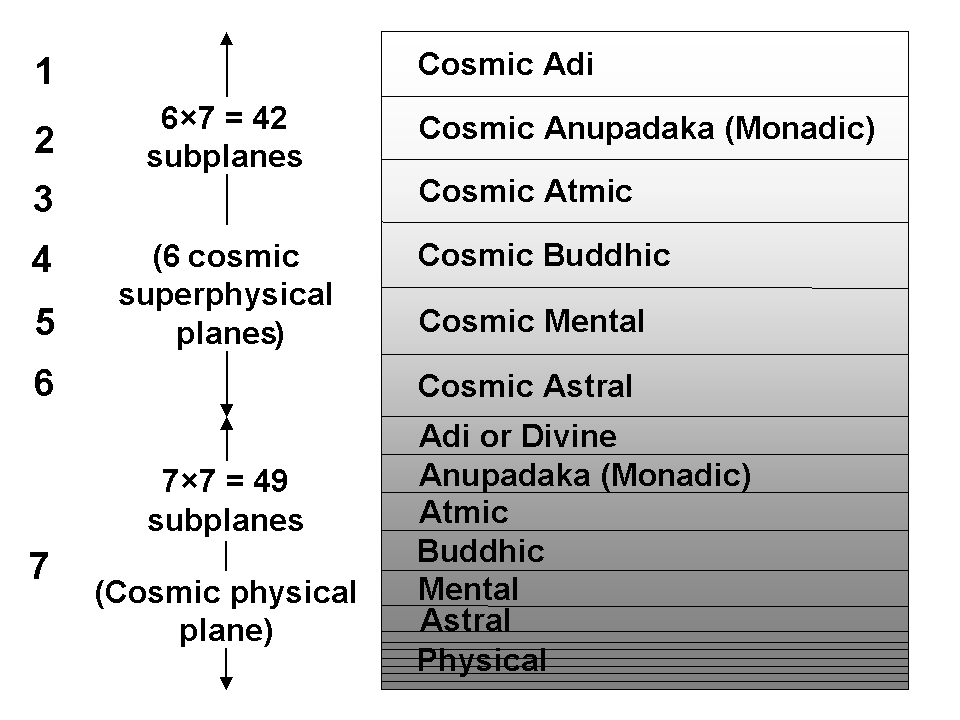The seven cosmic planes of consciousness.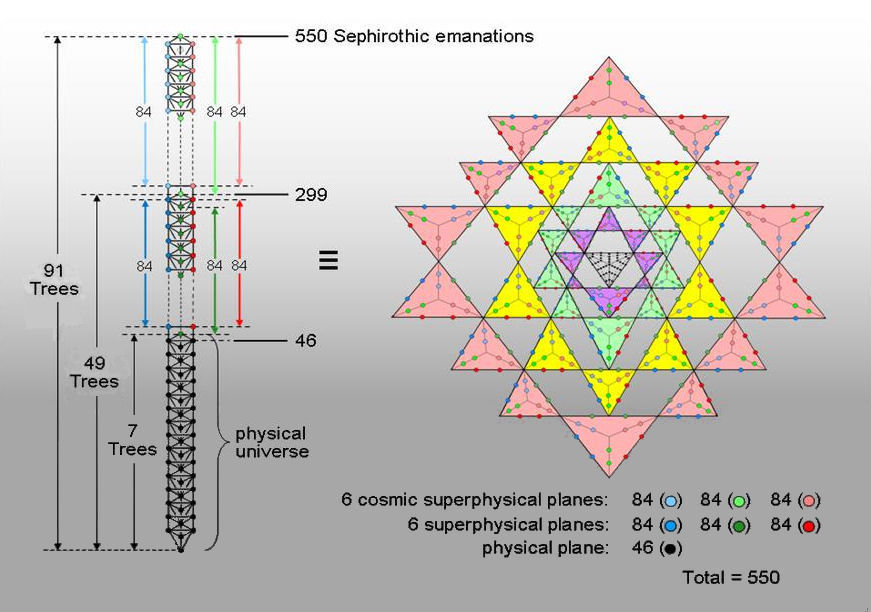Correspondence between the Cosmic Tree of Life and the 3-dimensional Sri Yantra.

There are 504 SLs in the 91 Trees of Life in CTOL down to the top of the 7-tree that maps the physical plane (26-dimensional space-time). The 84 Trees above it comprise the 42 Trees mapping the 42 subplanes of the six cosmic superphysical planes and the 42 analogous Trees that map the 42 subplanes of the six superphysical planes. The 252 SLs down to the top of the 49-tree mapping the 49 subplanes of the cosmic physical plane consist of three sets of 84 SLs. The 84 light red SLs span the Pillar of Mercy, the 84 light blue SLs span the Pillar of Judgement and the 84 light green SLs span the central Pillar of Equilibrium. There are three more similar sets of dark red, dark blue & dark green SLs down to the top of the 7-tree. Hence, 168 light & dark green SLs line the central pillar down to this point. This is how the number value 168 of Cholem Yesodoth, the Mundane Chakra of Malkuth, specifies that section of CTOL that is its Malkuth level, namely, the 7-tree, or physical plane. Forty-six more black SLs in the 7-tree extend to the lowest point of CTOL. Each of the seven cosmic planes expresses one of the seven Sephiroth of Construction, as do the seven subplanes comprising each cosmic plane, the seven planes of the cosmic physical plane and the seven subplanes of each of these planes.

Compare these properties with the 3-dimensional Sri Yantra when the 42 triangles are turned into Type A triangles and the central one is turned into a Type B triangle (for the definition of these two types of triangles, see Sacred Geometry/Tree of Life). The seven planes of consciousness have a one-to-one correspondence with the seven Sephiroth of Construction (physical↔Malkuth, astral↔Yesod, mental↔Hod, etc). The six superphysical planes are associated with the six Sephiroth of Construction other than Malkuth. In the tetractys, the latter are symbolized by the six hexagonal yods that lie on its edges, the central hexagonal yod denoting Malkuth. The 504 hexagonal yods that line the sides of the 126 tetractyses in the 42 triangles of the Sri Yantra are the counterpart of the 504 SLs belonging to the 84 Trees of Life mapping all superphysical realms of consciousness. The counterpart of the 46 SLs below the top of the 7-tree are the 46 black yods in the central triangle. The presence of the 504 hexagonal yods on sides of tetractyses would, of course, continue if it were the 2-dimensional version of the Sri Yantra that was being considered. So either version can be considered as equivalent to CTOL. However, the point at the centre of the central triangle is regarded in Tantra as the source of creation and this does not fit its symbolizing the bottom of CTOL — the opposite to this, being the final emanation of God. But then the central triangle symbolizes, according to Tantra, the trimûrti of Shiva, Brahma & Vishnu, and this is inconsistent with its identification with the physical universe mapped by the 7-tree. In order to retain the traditional meaning of the bindu point as standing outside all realms of consciousness, being their source, it does make a difference which version of the Sri Yantra is the correct counterpart of CTOL. Only its 3-dimensional form, which has this point hovering above the central triangle and the four layers of 42 triangles stacked on top of one another, is the right counterpart.

This encoding of CTOL in the Sri Yantra has its counterpart in a Type C dodecagon. The section Power of the polygons/dodecagon discusses the properties of this polygon. It is the single, polygonal representation of the archetypal pattern embodied in sacred geometries of western and eastern religions. The centre of the Type C dodecagon is surrounded by 504 yods: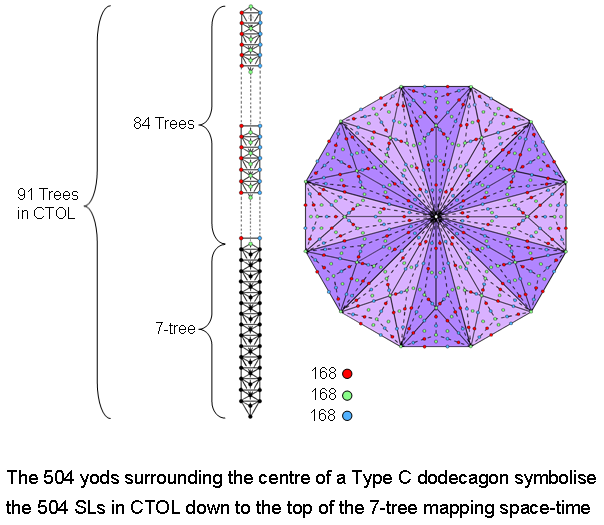There are 168 red SLs above the 7-tree on the left-hand Pillar of Judgement of the 91 Trees of Life representing CTOL. There are 168 blue SLs above the 7-tree on the right-hand Pillar of Mercy. 168 green SLs span the central Pillar of Equilibrium down to the top of the 7-tree. There are 14 green yods per sector of the Type C dodecagon that are either corners of tetractyses or hexagonal yods at their centres. The 9 tetractyses per sector have 14 sides, a red and a blue hexagonal yod lying on each one. Hence, the 504 yods surrounding the centre of the dodecagon comprise (12×14=168) yods that are either red, green or blue. They symbolise the three sets of 168 SLs lining the three pillars of CTOL down to the top of the 7-tree.

They form three sets of 168 yods (coloured red, green & blue) because each sector of the Type C dodecagon has nine tetractyses with 14 sides, each having a pair of red & blue hexagonal yods, and 14 green yods that are either corners of tetractyses or their centres, so that the (12×9=108) tetractyses contain (12×14=168) red hexagonal yods, 168 blue hexagonal yods and 168 green yods. These sets correspond to the 168 SLs on the three pillars of CTOL down to the top of the 7-tree mapping the physical plane (space-time). Hence, the Type C dodecagon is equivalent to the 3-dimensional Sri Yantra. Its centre corresponds to the central Type B triangle, whose 46 yods denote the 46 SLs below the top of the 7-tree. Its 504 yods correspond to the 504 hexagonal yods on the 252 sides of the 126 tetractyses making up the 42 Type A triangles of the Sri Yantra; they symbolise the 504 SLs in CTOL down to the top of the 7-tree.

The 91 overlapping Trees of Life in CTOL are composed of 3108* corners, sides & triangles, where

3108 = 14 + 34 + 54 + 74,

i.e., this number is the sum of the fourth powers of the first four odd integers. The geometrical composition of CTOL is determined by the Tetrad:Notice that 3108 = 444×7, where 7 is the fourth odd integer. As 3108 = 37×84, where 37 is the number of yods in the Type A hexagon, the fourth type of regular polygon, and 84 = 12 + 32 + 52 + 72, we see that the Tetrad determines the number quantifying the geometrical composition of CTOL, as well as its number (91) of Trees of Life, because the Type B hexagon has 91 yods: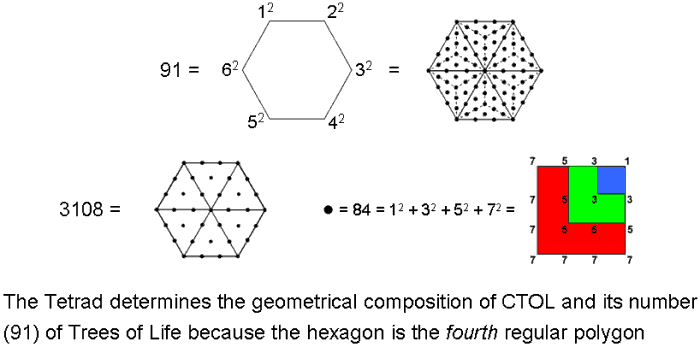The Tetrad also expresses the geometrical composition of the 2-dimensional Sri Yantra because 240 corners, sides & triangles surround its centre, where 240 = 10×24 = 1×2×3×4×(1+2+3+4). The physical plane (the space-time continuum) is mapped in CTOL by its lowest seven Trees of Life and in the Sri Yantra by its central triangle. As space-time, it is the Malkuth level of the 49-tree mapping the cosmic physical plane, the gematria number value of this Sephirah being 496, where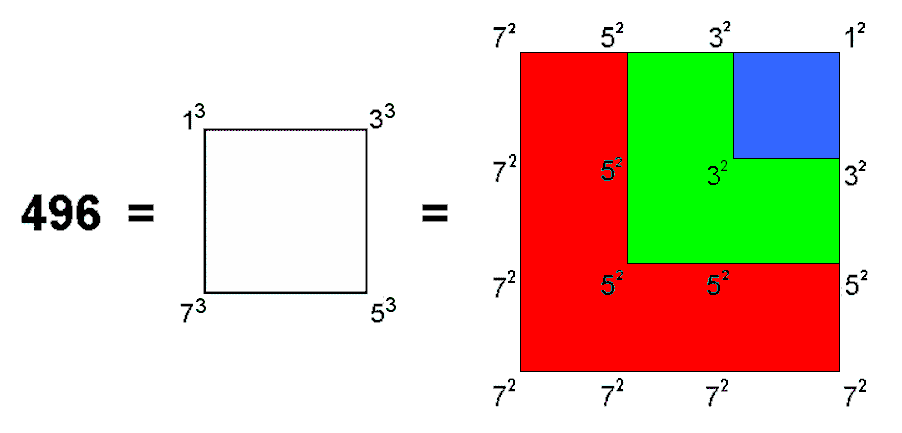It is no coincidence that this number is that found in 1984 by physicists Michael Green and Gary Schwarz to be the crucial dimension of a Yang-Mills gauge symmetry group governing the interactions of 10-dimensional superstrings that are free of quantum anomalies. It was then that research in theoretical physics encountered for the first time a mathematical aspect of the universe that is revealed by Kabbalah. But it is also revealed by the geometry of the Tree of Life, for this superstring parameter is embodied in the cosmic physical plane as the 496 yods either lying on or aligned with the Pillar of Equilibrium of 49 overlapping Trees of Life mapping its 49 subplanes when their triangles are tetractyses.** Making use of the formula proved in the first footnote, these 49 Trees are composed of (34×49 + 14 = 1680) points, lines & triangles. Here is a spectacular conjunction of the two most fundamental parameters of E8×E8 heterotic superstrings — one (496) referring to their forces described by the symmetries of E8×E8 and the other (1680), yet to be discovered by physicists, that refers to their 4-dimensional space-time structure in their subquark ground state, namely, the 1680 circular turns of each helical whorl of the UPA counted by C.W. Leadbeater when he observed it with micro-psi over a century ago. Here in the conjunction between two numbers referring to the same context is an undeniable meeting point of the mystical, the scientific and the paranormal. Provided he is honest, even the most die-hard sceptic towards the paranormal would admit that it is too unlikely that the simultaneous appearance of these two numbers (one scientifically based, the other paranormally obtained) in the Tree of Life representation of the cosmic physical plane might be due to chance. Yet the sceptic is forced to embrace the miraculously improbable in order to avoid believing what is anathema to him, namely, that remote viewing of the subatomic world is possible and was successfully achieved by Besant & Leadbeater. No one should be fooled by the sceptic's ideological disbelief in the paranormal forcing him to reject all common sense concerning what can be reasonably regarded as coincidence. When prima facie evidence such as this is rejected for highly implausible reasons, one cannot fail to conclude that an unscientific attitude of bias is at work.

* Proof: Number of corners of triangles in n overlapping Trees of Life = 6n + 4.
Number of sides of triangles in n overlapping Trees of Life = 16n + 6.
Number of triangles in n overlapping Trees of Life = 12n + 4.
Number of corners, sides & triangles in n overlapping Trees of Life = 6n + 4 + 16n + 6 + 12n + 4 = 34n + 14. For n = 91, this number is 3108.
** Proof: The number of yods on the Pillar of Equilibrium from Malkuth of the 1-tree up to (but not including) Tiphareth of the 1-tree = 9 (see here). The quadrilateral Faces of successive Trees have 10 yods per Face that are on, or aligned with, this pillar (see here). For n overlapping Trees, there are (n−1) Faces between the lowest Face of the 1-tree and the highest Face of the nth Tree, which contain 7 such yods from its Tiphareth to its Kether. Number of yods on, or aligned with, the Pillar of Equilibrium in n overlapping Trees = 9 + 10(n−1) + 7 = 10n + 6. For n = 49, this is 496.

 << Previous      2    Next >>

Home